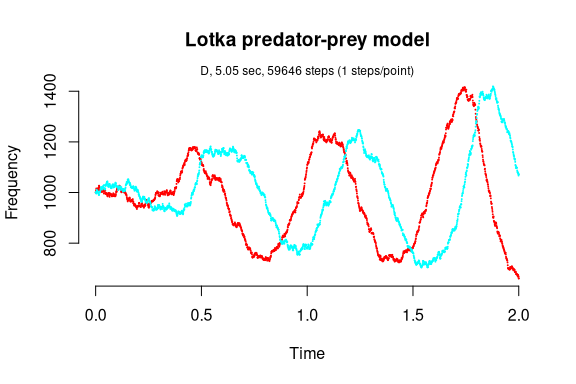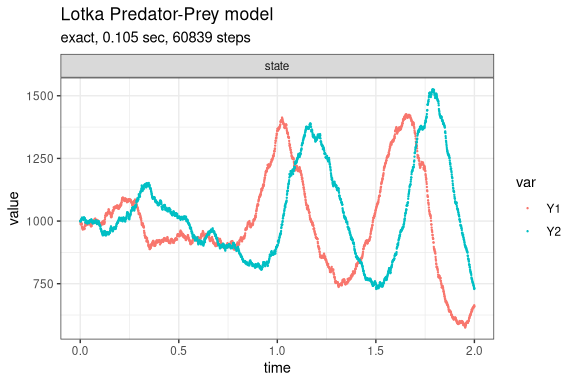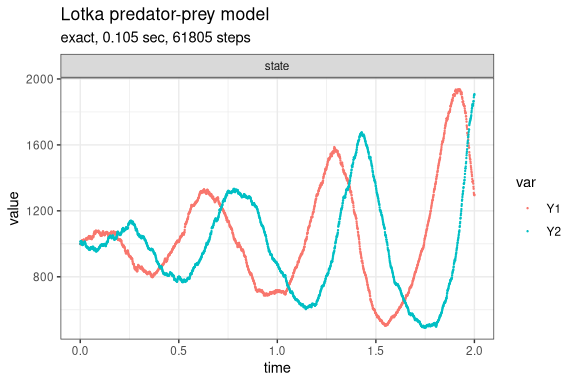# Converting from GillespieSSA to GillespieSSA2

In this vignette, we will show how to upgrade the Lotka-predator-prey model from GillespieSSA to GillespieSSA2. First, have a look at the GillespieSSA vignette:

• CRAN Vignette
• vignette("lotka_predator_prey", package = "GillespieSSA")

The model consists of the following objects:

library(GillespieSSA)
parms <- c(c1 = 10, c2 = .01, c3 = 10)
tf <- 2                                        # Final time
simName <- "Lotka predator-prey model"         # Name
x0 <- c(Y1=1000, Y2=1000)
nu <- matrix(c(+1, -1, 0, 0, 1, -1), nrow = 2, byrow = TRUE)
a  <- c("c1*Y1", "c2*Y1*Y2","c3*Y2")
out <- GillespieSSA::ssa(
x0 = x0,
a = a,
nu = nu,
parms = parms,
tf = tf,
method = ssa.d(),
simName = simName,
censusInterval = .001,
verbose = FALSE,
consoleInterval = 1
)
ssa.plot(out, show.title = TRUE, show.legend = FALSE)In order to port these objects, it is preferable to use the updated notation from the respective GillespieSSA2 vignette:

• CRAN Vignette
• vignette("lotka_predator_prey", package = "GillespieSSA2")
library(GillespieSSA2)
sim_name <- "Lotka Predator-Prey model"
params <- c(c1 = 10, c2 = .01, c3 = 10)
final_time <- 2
initial_state <- c(Y1 = 1000, Y2 = 1000)
reactions <- list(
reaction("c1 * Y1",      c(Y1 = +1)),
reaction("c2 * Y1 * Y2", c(Y1 = -1, Y2 = +1)),
reaction("c3 * Y2",      c(Y2 = -1))
)
out <- GillespieSSA2::ssa(
initial_state = initial_state,
reactions = reactions,
params = params,
final_time = final_time,
method = ssa_exact(),
census_interval = .001,
verbose = FALSE,
sim_name = sim_name
)
plot_ssa(out)However, if you want to use the GillespieSSA1 objects directly, you can also transform these programmatically. The interface of GillespieSSA2 is very similar, it only requires you to use different parameter names, and combine the state-change matrix nu and the propensity functions a into one list of reactions.

out <-
GillespieSSA2::ssa(
initial_state = x0,
reactions = port_reactions(x0 = x0, a = a, nu = nu),
params = parms,
method = ssa_exact(),
final_time = tf,
census_interval = .001,
verbose = FALSE,
sim_name = simName
)
print(out\$stats)
##   method                  sim_name sim_time_exceeded all_zero_state
## 1  exact Lotka predator-prey model              TRUE          FALSE
##   negative_state all_zero_propensity negative_propensity walltime_exceeded
## 1          FALSE               FALSE               FALSE             FALSE
##   walltime_elapsed num_steps  dtime_mean     dtime_sd firings_mean firings_sd
## 1        0.1053002     61805 3.23602e-05 1.378919e-08            1          0
plot_ssa(out)